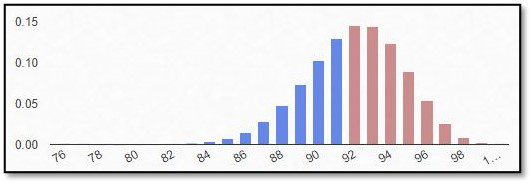# BlogBiostatistics

### What is the binominal distribution?

If an outcome variable that can be specified in two response possibilities only, data can be analyzed as binary based on a binomial distribution, where each observation of a defined number of “experiments” represents one of the two outcomes, and the probability of success is the same for each observation.
In this part of our blog series accompanying our #video #series “5 Minutes Statistics for Clinical Trials” we explain what a #binomial #distribution reflects and what are its characteristics.

### What is the difference between absolute, relative, and cumulative frequencies of occurrence?

Descriptive statistics present a good first overview of the data. The frequency of occurrence, as part of descriptive statistics, provides information that is easy to

### What is the scatter, dispersion and shape of a distribution?

When presenting the data descriptively, the distribution of single variables can include the following parameters: Scatter parameters such as the mean, median, and mode; dispersion